# Notation of matrices

### Notation of Matrices

One of the most important tools used throughout linear algebra, and thus one of the key points to learn on this course, is matrix mathematics. Due to linear algebra being all about finding the solutions to systems of linear equations, matrix math and the study of vector spaces become a tool to represent and orderly solve such systems in an orderly and intuitive fashion.

The lesson of today focuses on the introduction of this new kind of mathematical information arrangement: the matrix (plural: matrices). On our lesson we will introduce the concept of a matrix, how can it be operated on mathematically, and then there will be a little introduction preparing you for the next lessons on this course, where you will learn how useful matrices are and the methods to solve mathematical equations with them.

### What is a matrix?

In mathematics, a matrix is an ordered list of numbers put in a rectangular bracket. This list can also be called a rectangular array, and the reason for it, is that its elements can represent different coefficient terms or coefficients of variables depending on their location within the bracket box.

A matrix provides an orderly fashion to display an array of information. For example: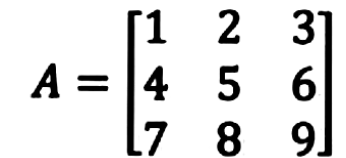Equation 1: Example of a matrix

Notice the rectangular brackets are NOT just simple straight lines surrounding the array of numbers, it is important to note this since there is a different operation which can be obtain from a matrix called the determinant, which happens to have a notation very similar to the matrix itself, but instead of rectangular brackets it has straight lines around. Just remember, if the numbers are inside a "box" is a bracket matrix, if the numbers are inside two long straight lines then is a determinant. We will talk about the determinant of a 2x2 matrix and of the 3x3 matrix, along with the properties of determinants in lessons much later on this course. For now, we will concentrate on the basics of matrix notations to continue on their proper operations.

Before we learn the matrix definition let us talk about the history of this word and how it makes sense to have such a notation among math: It is no wonder how the term "matrix" in itself has been cleverly used throughout history not only among popular culture with different connotations depending on the epoch, but also through different academic areas of study from mathematics to different branches of science.

The word "matrix", from its latin etymology, means "womb" and it is used not only in math but also in areas such as biology, where is the term used to name the tissue on living organisms cells where more specialized materials (those structures with higher or more specific functions) are lodged. You can also found the term matrix being used in chemical analysis and experimentation, where the word describes all of the rest of the content in a sample which is not the particular material of interest. In geology, a matrix refers to the compact material without a characteristic shape in which well defined specimens of rock formation or crystals are embedded.

If we pay attention to all of these usages of the word throughout areas of natural science, we can find a pattern where in general we define matrix as an environment in which information of interest (be it structures or systems) is lodged, fixed or contained, and ready for us to study or use it. The same goes for matrix algebra, in mathematics we use matrix notation to organize systems of values coming from different geometrical planes. This will become obvious as we continue with our linear algebra lessons, and so we will just be mentioning this for now so you can start having an idea of what matrices are all about. On the next lessons where you will be solving systems of linear equations by graphing, and then learning how to represent a linear system as a matrix, you will be able to directly observe what we have been talking about on the usefulness and meaning of matrices as "boxes" of information.

### Matrix dimensions

The dimensions of a matrix define its size and it basically refers to the number of rows and columns inside the matrix. For example, if the matrix has m rows and n columns, then we say that the dimensions of a matrix are m by n.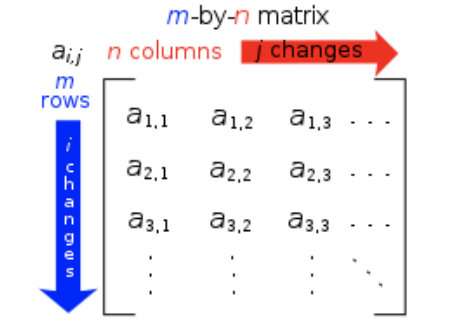Figure 1: Dimensions of a matrix explained

In this way, each entry in a matrix gets called a matrix element which we can denote with subindexes. For example, if we have a m by n matrix A. Then we say that the matrix element $a_{3,2}$ is the entry in the third row and second column.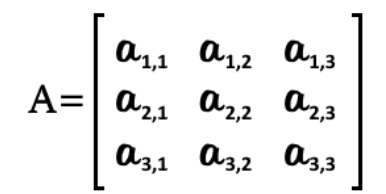Equation 2: Matrix elements

Let us work through a a few examples next:

Example 1

Determine the dimensions of the following matrices: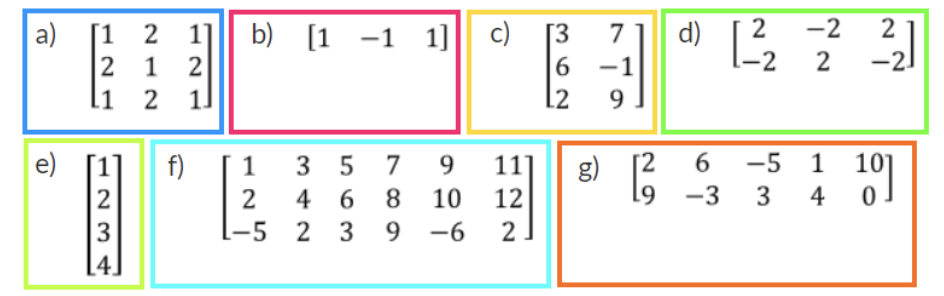Equation 3: Examples of matrices

And so the dimensions for each matrix are:

1. 3 x 3 = 3 rows and 3 columns
2. 1 x 3 = 1 row and 3 columns
3. 3 x 2 = 3 rows and 2 columns
4. 2 x 3 = 2 rows and 3 columns
5. 4 x 1 = 4 rows and 1 column
6. 3 x 6 = 3 rows and 6 columns
7. 2 x 5 = 2 rows and 5 columns

Example 2

Find the matrix elements (or values) asked below from the following matrix: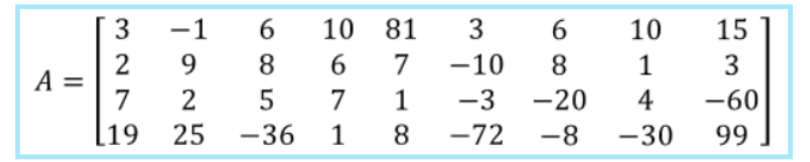Equation 4: 4 x 9 Matrix
1. Element $a_{1,1}$ = 3
2. Element $a_{2,3}$ = 8
3. Element $a_{4,6}$ = -72
4. Element $a_{4,9}$ = 99
5. Value of $\frac{a_{2,5}}{a_{4,9}} = \frac{7}{99}$ = 0.0707
6. Value of 2$(a_{3,7})$ = 2(-20) = -40
7. Value of $(a_{2,4})^{2} = (6)^{2}$= 36
8. Value of 2$(a_{4,2})$ + 5 = 2(25) + 5 = 55
9. Value of $\frac{a_{1,9}}{3} = \frac{15}{3}$ = 5
10. Value of $a_{3,5} + a_{1,1}$ = 1 + 3 = 4

After learning how the dimension of a matrix provides information on the characteristics of the array notation and knowing what the elements in a matrix are, it is important to recognize there are a few different types of matrices:

• Square matrices:

When a matrix has the same amount of columns and rows, we call this matrix a square matrix. A square matrix then, is a mxn matrix in which m=n, and thus, its box notation does look like a square box since the elements inside the matrix bracket are the same amount on each side. Since in this case m equals n, we usually refer to square matrices dimensions simply as nxn. Equation 2 shows the notation of a square matrix with dimensions 3x3.

• Diagonal matrices:

Although diagonal matrices can refer to matrices with any combination of dimensions, the term is more often used when talking about specific types of square matrices. The reason for this is that a diagonal matrix will be easily identified by the difference between the type of elements it has in its main diagonal, versus the rest of the array.

The main diagonal of a matrix is the diagonal array of numbers which start from its uppermost left corner progressing downwards towards the right side until you get to the lowest possible row. For a square matrix, the main diagonal goes from the upper left corner to the bottom right corner. If we take equation 2 as our provided matrix, the main diagonal of this matrix contains the elements: $a_{1,1}, a_{2,2}$ and $a_{3,3}$. For all matrices, the main diagonal is the array of elements with subindexes where m equals n, and so, the main diagonal of any matrix is the array of elements such that: $a_{1,1}, a_{2,2}, a_{3,3}... a_{n,n}$ where m = n.

And so, a diagonal matrix is that in which only the elements inside its main diagonal are different to zero.

• Identity matrix:

Identity matrices are square matrices by definition which contain only values of 1 in their main diagonal. Identity matrices are denoted as $I_{n}$ where n represents the dimension of the matrix nxn. Such matrices are important in vector matrix notation since they provide a way to clearly discern between the terms of different variables in collections of vectors written as matrix, although we will continue with this topic in a deeper manner in later lessons, it is important for you to know that the identity matrix notation provides a base for unit vector notation in linear algebra, and thus, an identity matrix can be called a unit matrix too.

All identity matrices are diagonal matrices, and any multiples of them will be too because the only values affected by a scalar multiplication are those on the main diagonal (since the rest of them are zeros, any number multiplied by zero equals to zero, and so the zeros remain unchanged).

• Zero matrix:

A zero matrix is that which has only zeros as its elements. Zero matrix notation is probably the simplest, since it does not matter the dimensions of the matrix in question, as long as all of the elements inside it are zeros, you have a zero matrix. Zero matrices may be known as null matrices too.

All of these will be studied in depth throughout the lessons of this course since they will be most useful through different operations or methods while solving linear systems of equations.

Example 3

Identify which type of matrix is each of the ones in the next list, write down their dimensions and the characteristics that allowed you to identify it: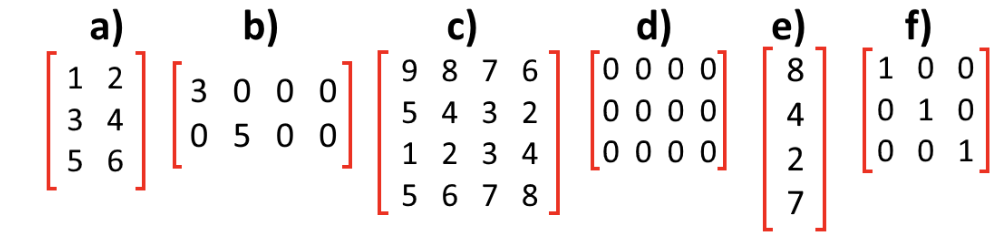Equation 5: Different types of matrices

And so to identify each of the matrices above:

1. This is a 3 x 2 matrix, which means that it has three rows and two columns as it can be easily observed.
2. This matrix is a diagonal matrix, since it has a different amount of rows and columns, we refer to these types of matrices as "rectangular diagonal" matrices. The dimensions of matrix b) are 2 x 4.
3. This is a square matrix of dimensions 4 x 4 (4 rows and 4 columns).
4. Matrix d) is a zero matrix since all of its entries are zeros.
5. Matrix e) is a column matrix with dimensions 4 x 1 (all column matrices consist of one column and are also known as vector matrices).
6. This matrix is an identity matrix $I_{3}$ which means that the dimension of matrix f) is 3, or 3 x 3 (3 rows and 3 columns).

Before we finalize this lesson with an introduction on the rules that apply to basic matrix operations we would like to recommend you to visit these notes on matrix notation and operations if you want to continue looking at examples of what we have learned so far on this course. Another nice and straightforward article you can visit is this one on matrix notation, where you can find a few more examples in a simplistic form.

### Matrix rules

For this section we will have a brief introduction to the matrix rules for operations such as addition and multiplication. These types of operations will be covered in depth and with examples later throughout this Linear Algebra course, but it is important to have a basic idea on how the matrix size and how many rows vs columns a matrix has provides guidelines on what operations can be done with such matrix or not.

• Addition / subtraction:

The addition (and thus subtraction) of two matrices is a very simple operation to compute. The rule is that as long as you have two matrices with the same amount of rows and columns, you can add (or subtract) them. The resultant matrix will have the same amount of rows and columns as the two original matrices and the operation is simply done by adding the elements with the same subindexes from each matrix and positioning the result in the element with the same subindex in the resultant matrix. Take a look at figure 2 where you can observe the general rule for adding matrices: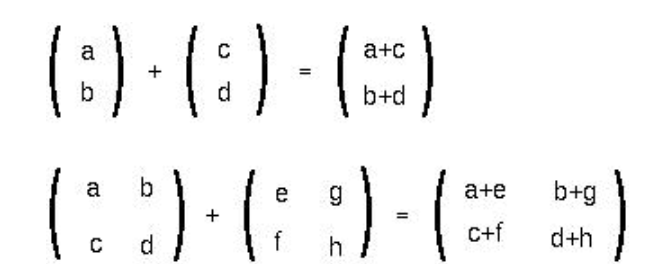Figure 2: Matrix addition
• Multiplication: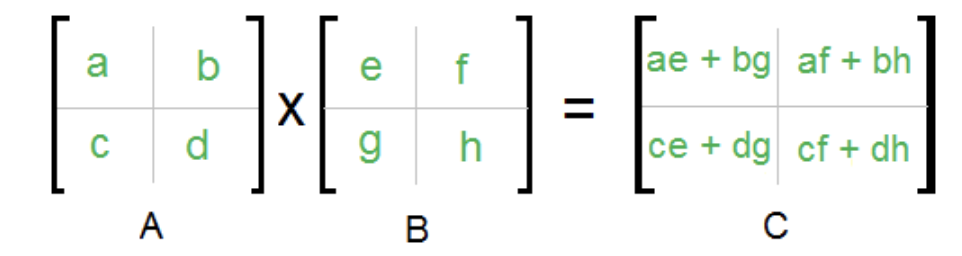Figure 3: Rule for matrix multiplication

In order to multiply two matrices, the first matrix must have the same amount of columns as the second matrix has rows. The product of this multiplication, will be a new matrix with dimensions equal to the amount of rows in the first matrix by the amount of columns found in the second matrix. And so, this means that for two matrices $A = m_{1} \times n_{1}$ and $B = m_{2} \times n_{2}$ to be multiplied, $n_{1}$ must be equal to $m_{2}$ and the resulting matrix will have the dimensions of $C = m_{1} \times n_{2}$. This can be observed in the following figure: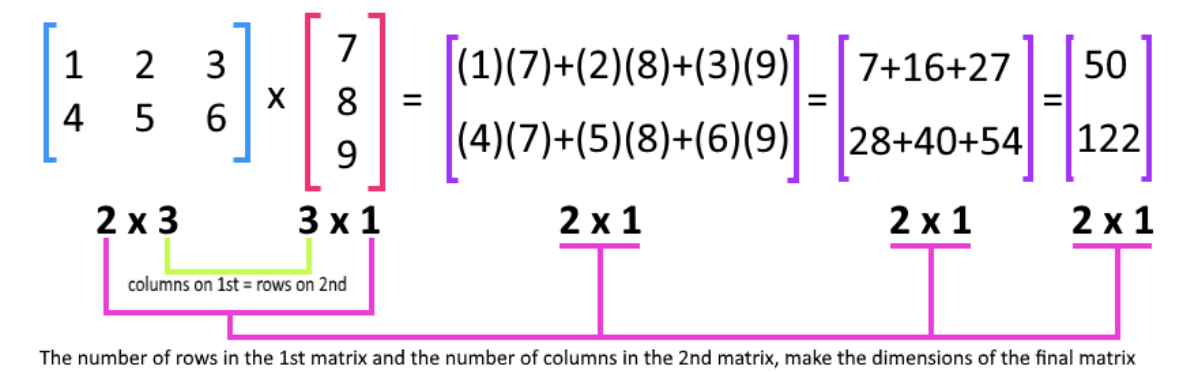Figure 4: Matrix Multiplication explained

And so, as you may have already noticed, matrix multiplication is NOT commutative: you cannot change the order of the matrices being multiplied and obtain the same result, actually, you won't be able to multiply them due to the fact that, unless they are square matrices, the first matrix will not contain the same amount of columns as the amounts of rows in the second one, making the multiplication not possible.

This is all for our topic of today, as you can see, this lesson serves as an introduction to the algebraic notation we will be using throughout most of this course and any course on Linear Algebra. Make sure you understand the examples so you can be ready to the next lesson.

### Notation of matrices

In this section, we will be learning about the dimensions of a matrix, as well as finding the matrix element within a matrix. Dimensions of a matrix are determined by the number of rows and columns in the matrix. We generally write the dimensions to be (# of rows) x (# of columns). A matrix element is an entry in the matrix. If you see the subscript of the letter, then that subscript will tell you which entry we are talking about in the matrix. For example, if the subscript is (4,6), then we are looking for the entry in the 4th row and 6th column.

#### Lessons

A matrix is a list of numbers put in a rectangular bracket.

The dimensions of a matrix are the number of rows and columns of the matrix. For example, if the matrix has m rows and n columns, then we say that the dimensions matrix is m by n.

Each entry in the matrix is called a matrix element. Let the matrix be called A. Then we say that the matrix element $a_{4,6}$ is the entry in the 4th row and 6th column.

• Introduction
Notation of Matrices Overview:
a)
What is a matrix and the dimension of a matrix?

b)
Matrix elements

• 1.
The Dimensions of a Matrix
Determine the dimensions of the following matrices:
a)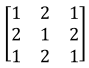b)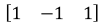c)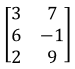d)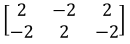e)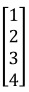f)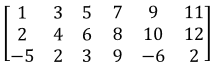g)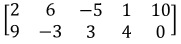• 2.
Matrix Elements
Find the matrix element (or value) of the following matrix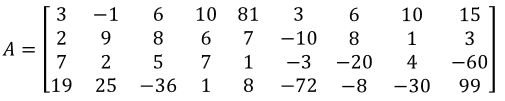a)
$a_{1,1}$

b)
$a_{2,3}$

c)
$a_{4,6}$

d)
$a_{4,9}$

e)
$\frac{a_{2,5}}{a_{4,9}}$

f)
$2(a_{3,7})$

g)
$(a_{2,4})^2$

h)
$2(a_{4,2})+5$

i)
$\frac{a_{1,9}}{3}$

j)
$a_{3,5}+a_{1,1}$